Open in App
Not now

# Maximize score to fill Matrix when score of a step is count of adjacent filled cells

• Last Updated : 23 Jan, 2023

Given two integers N and M that denotes the number of rows and columns of a matrix that is initially empty, the task is to fill all the empty cells and find the maximum score while filling them when the score to fill a cell is the same as the count of adjacent filled cells.

Examples:

Input: N=2, M=3
Output: 7
Explanation:  Matrix after applying 3 operations: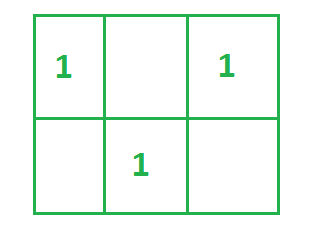Matrix after applying three operations

Till 3 operations sum is zero. Because each cell having 1 as element has no adjacent cell having element 1. Matrix after 3 more operations: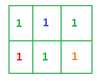Matrix in 4th, 5th and 6th operation

4th Cell has element 1 in red color has 2 adjacent cells(up and right) having 1 . Score till here is 2.
2nd Cell has element 1 in blue color has 3 adjacent cells(left, right, down) having 1. Score till here is 2+3=5.
6th Cell has element 1 in Orange color has 2 adjacent cells(left, up) having 1. Score till here is 5+2=7.
Hence, Total maximum score that can obtain is 7.

Input: N = 5, M = 7
Output: 58

Approach: The problem can be solved based on the following idea:

Put 1 on cells of matrix in such a way that it looks like chessboard of elements 1 and empty cells. Then just put 1 at empty cells and count adjacent cell having 1. For more clarification, See the illustration of approach below.

Illustration of approach:

If N = 4, M = 4

initially Matrix will be: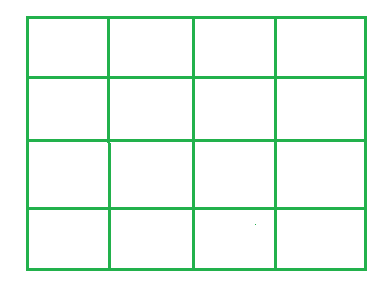Initial matrix

For making chessboard like pattern of 1, Both the index of matrix should be odd or even. On traversing process on matrix we will skip these cells, Because they are not contributing in increasing score. After applying 8 operations and making matrix like chessboard of element 1: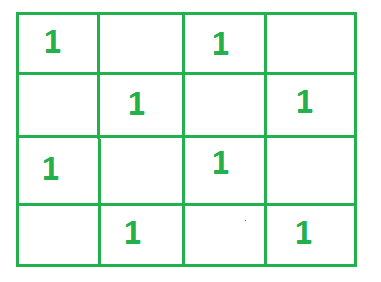Matrix applying 8 operations(from 1 to 8)

Now,  make 8 more operations one by one, put 1 at empty cells of matrix and count number of adjacent elements while putting 1 in each of these operations.

In image below next 8 operations are shown and adjacent cells are showed by arrows.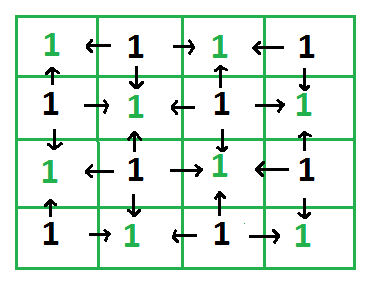matrix after applying more 8 operations(from 9 to 16)

In the image above, 8 black 1s are put one by one in operations from 9 to 16 and adjacent cells are represented by arrows. Formally, Each arrow can be considered as 1 score, There are total 24 arrows. Hence 4*4 matrix will have maximum possible score as 24, in exactly 16 operations.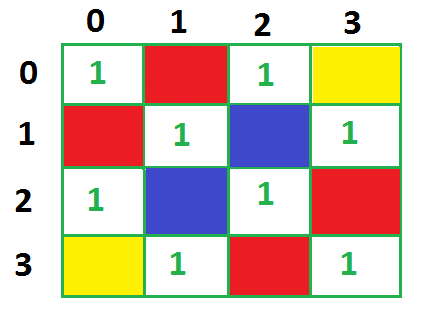Observations

From the above image it can be seen,

• If the empty cell is at any corner suppose (yellow color in above picture) then it will contribute 2 to total score.
• Those cells which are at borders (red cells) will contribute 3 to total score
• Rest of the cell (blue colored) will contribute 4 in total score.

Follow the below steps for applying the brute force approach.

• Traverse on the matrix using two nested Loops.
• If both indices are odd or even, Then skip those cells of the matrix.
• Initialize a variable total_sum, and add 2, 3 or 4 to total_sum by testing empty cell is at the corner, border, or at other position than these two, by using discussed approach above.
• Print value of total_sum

Below is the implementation of the above approach.

## C++

 `// C++ implementation``#include ``using` `namespace` `std;` `// function to check both indices provided are odd or not``bool` `is_Odd(``int` `a, ``int` `b)``{``  ``if` `((a & 1) == 1 && (b & 1) == 1) {``    ``return` `true``;``  ``}``  ``return` `false``;``}` `// function to check if both indices are even or not``bool` `is_Even(``int` `a, ``int` `b)``{``  ``if` `((a & 1) != 1 && (b & 1) != 1) {``    ``return` `true``;``  ``}``  ``return` `false``;``}` `int` `main()``{` `  ``// Input value of n and m``  ``int` `n = 2, m = 3;` `  ``// Variable to store total maximum score``  ``long` `total_score = 0;` `  ``// Condition when either row or column is equal to 1``  ``if` `(n == 1 || m == 1) {` `    ``// If (row||col) = 1, Then total score will be``    ``// absolute difference of (m-n)``    ``total_score = ``abs``(n - m);``  ``}` `  ``// Loop for Traversing on rows``  ``for` `(``int` `i = 0; i < n; i++) {` `    ``// Loop for traversing on columns``    ``for` `(``int` `j = 0; j < m; j++) {` `      ``// If cells indices (i, j) are odd or even``      ``// Then skipping those cells``      ``if` `(is_Odd(i, j) || is_Even(i, j)) {``        ``continue``;``      ``}` `      ``// Empty cells will execute in else part``      ``// below``      ``else` `{` `        ``// Condition for corner empty cases``        ``if` `((i == 0 && j == 0)``            ``|| (i == (n - 1) && j == (m - 1))``            ``|| (i == (n - 1) && j == (0))``            ``|| (i == (0) && j == (m - 1))) {` `          ``// Corner empty cell contributes 2``          ``// in total_score``          ``total_score += 2;``        ``}` `        ``// Condition for border empty cells``        ``else` `if` `(i == 0 || i == (n - 1) || j == 0``                 ``|| j == (m - 1)) {``          ``// Border empty cell contributes 2``          ``// in total_score``          ``total_score += 3;``        ``}` `        ``// Rest of the cells except border and``        ``// corner(blue colored)``        ``else` `{` `          ``// Will contribute 4 in total_score``          ``total_score += 4;``        ``}``      ``}``    ``}``  ``}` `  ``// Printing value of total_score variable``  ``cout << (total_score);` `  ``return` `0;``}` `// This code is contributed by ksam24000`

## Java

 `// Java code to implement the approach``import` `java.io.*;``import` `java.lang.*;``import` `java.util.*;` `class` `GFG {``    ``// function to check both indices provided are odd or not``    ``static` `boolean` `is_Odd(``int` `a, ``int` `b)``    ``{``        ``if` `((a & ``1``) == ``1` `&& (b & ``1``) == ``1``) {``            ``return` `true``;``        ``}``        ``return` `false``;``    ``}` `    ``// function to check if both indices are even or not``    ``static` `boolean` `is_Even(``int` `a, ``int` `b)``    ``{``        ``if` `((a & ``1``) != ``1` `&& (b & ``1``) != ``1``) {``            ``return` `true``;``        ``}``        ``return` `false``;``    ``}` `    ``public` `static` `void` `main(String args[])``    ``{` `        ``// Input value of n and m``        ``int` `n = ``2``, m = ``3``;` `        ``// Variable to store total maximum score``        ``long` `total_score = ``0``;` `        ``// Condition when either row or column is equal to 1``        ``if` `(n == ``1` `|| m == ``1``) {` `            ``// If (row||col) = 1, Then total score will be``            ``// absolute difference of (m-n)``            ``total_score = Math.abs(n - m);``        ``}` `        ``// Loop for Traversing on rows``        ``for` `(``int` `i = ``0``; i < n; i++) {` `            ``// Loop for traversing on columns``            ``for` `(``int` `j = ``0``; j < m; j++) {` `                ``// If cells indices (i, j) are odd or even``                ``// Then skipping those cells``                ``if` `(is_Odd(i, j) || is_Even(i, j)) {``                    ``continue``;``                ``}` `                ``// Empty cells will execute in else part``                ``// below``                ``else` `{` `                    ``// Condition for corner empty cases``                    ``if` `((i == ``0` `&& j == ``0``)``                        ``|| (i == (n - ``1``) && j == (m - ``1``))``                        ``|| (i == (n - ``1``) && j == (``0``))``                        ``|| (i == (``0``) && j == (m - ``1``))) {` `                        ``// Corner empty cell contributes 2``                        ``// in total_score``                        ``total_score += ``2``;``                    ``}` `                    ``// Condition for border empty cells``                    ``else` `if` `(i == ``0` `|| i == (n - ``1``)``                             ``|| j == ``0` `|| j == (m - ``1``)) {``                        ``// Border empty cell contributes 2``                        ``// in total_score``                        ``total_score += ``3``;``                    ``}` `                    ``// Rest of the cells except border and``                    ``// corner(blue colored)``                    ``else` `{` `                        ``// Will contribute 4 in total_score``                        ``total_score += ``4``;``                    ``}``                ``}``            ``}``        ``}` `        ``// Printing value of total_score variable``        ``System.out.println(total_score);``    ``}``}`

## Python3

 `# function to check both indices provided are odd or not``def` `is_odd(a, b):``    ``if` `(a & ``1``) ``=``=` `1` `and` `(b & ``1``) ``=``=` `1``:``        ``return` `True``    ``return` `False` `# function to check if both indices are even or not``def` `is_even(a, b):``    ``if` `(a & ``1` `!``=` `1``) ``and` `(b & ``1` `!``=` `1``):``        ``return` `True``    ``return` `False` `# Input values of n and m``n ``=` `2``m ``=` `3` `# variables to store the total maximum score``total_score ``=` `0` `# condition when either row or column is equal to 1``if` `n ``=``=` `1` `or` `m ``=``=` `1``:``    ``# If row||col = , then total score will be absolute difference``    ``# of m-n``    ``total_score ``=` `abs``(n``-``m)` `# loop for traversing on rows``for` `i ``in` `range``(n):``  ` `    ``# loop for traversing the columns``    ``for` `j ``in` `range``(m):``      ` `        ``# if cell indices (i, j) are odd or even``        ``# then skipping those cells``        ``if` `is_odd(i, j) ``or` `is_even(i, j):``            ``continue` `        ``# Empty cells will execute in else part``        ``# below``        ``# Condition for corner empty cases``        ``if` `((i ``=``=` `0` `and` `j ``=``=` `0``) ``or` `(i ``=``=` `(n ``-` `1``) ``and` `j ``=``=` `(m ``-` `1``)) ``or` `(i ``=``=` `(n ``-` `1``)``and` `j ``=``=` `(``0``))``or` `(i ``=``=` `(``0``) ``and` `j ``=``=` `(m ``-` `1``))):``          ``# Corner empty cell contributes 2``          ``# in total_score``          ``total_score ``+``=` `2`  `        ``# Condition for border empty cells``        ``elif` `(i ``=``=` `0` `or` `i ``=``=` `(n ``-` `1``) ``or` `j ``=``=` `0` `or` `j ``=``=` `(m ``-` `1``)):``          ` `          ``# Border empty cell contributes 2``          ``# in total_score``          ``total_score ``+``=` `3` `        ``# Rest of the cells except border and``        ``# corner(blue colored)``        ``else``:``            ``# Will contribute 4 in total_score``            ``total_score ``+``=` `4` `    ``# Printing value of total_score variable``print``(total_score)` `# This code is contributed by Aditya Sharma`

## C#

 `// C# code to implement the approach` `using` `System;` `public` `class` `GFG {` `    ``// function to check both indices provided are odd or``    ``// not``    ``static` `bool` `is_Odd(``int` `a, ``int` `b)``    ``{``        ``if` `((a & 1) == 1 && (b & 1) == 1) {``            ``return` `true``;``        ``}``        ``return` `false``;``    ``}` `    ``// function to check if both indices are even or not``    ``static` `bool` `is_Even(``int` `a, ``int` `b)``    ``{``        ``if` `((a & 1) != 1 && (b & 1) != 1) {``            ``return` `true``;``        ``}``        ``return` `false``;``    ``}` `    ``static` `public` `void` `Main()``    ``{` `        ``// Input value of n and m``        ``int` `n = 2, m = 3;` `        ``// Variable to store total maximum score``        ``long` `total_score = 0;` `        ``// Condition when either row or column is equal to 1``        ``if` `(n == 1 || m == 1) {` `            ``// If (row||col) = 1, Then total score will be``            ``// absolute difference of (m-n)``            ``total_score = Math.Abs(n - m);``        ``}` `        ``// Loop for Traversing on rows``        ``for` `(``int` `i = 0; i < n; i++) {` `            ``// Loop for traversing on columns``            ``for` `(``int` `j = 0; j < m; j++) {` `                ``// If cells indices (i, j) are odd or even``                ``// Then skipping those cells``                ``if` `(is_Odd(i, j) || is_Even(i, j)) {``                    ``continue``;``                ``}` `                ``// Empty cells will execute in else part``                ``// below``                ``else` `{` `                    ``// Condition for corner empty cases``                    ``if` `((i == 0 && j == 0)``                        ``|| (i == (n - 1) && j == (m - 1))``                        ``|| (i == (n - 1) && j == (0))``                        ``|| (i == (0) && j == (m - 1))) {` `                        ``// Corner empty cell contributes 2``                        ``// in total_score``                        ``total_score += 2;``                    ``}` `                    ``// Condition for border empty cells``                    ``else` `if` `(i == 0 || i == (n - 1)``                             ``|| j == 0 || j == (m - 1)) {``                        ``// Border empty cell contributes 2``                        ``// in total_score``                        ``total_score += 3;``                    ``}` `                    ``// Rest of the cells except border and``                    ``// corner(blue colored)``                    ``else` `{` `                        ``// Will contribute 4 in total_score``                        ``total_score += 4;``                    ``}``                ``}``            ``}``        ``}` `        ``// Printing value of total_score variable``        ``Console.WriteLine(total_score);``    ``}``}` `// This code is contributed by lokeshmvs21.`

## Javascript

 `// Javascript implementation` `// function to check both indices provided are odd or not``function` `is_Odd(a,b)``{``  ``if` `((a & 1) == 1 && (b & 1) == 1) {``    ``return` `true``;``  ``}``  ``return` `false``;``}` `// function to check if both indices are even or not``function` `is_Even(a,b)``{``  ``if` `((a & 1) != 1 && (b & 1) != 1) {``    ``return` `true``;``  ``}``  ``return` `false``;``}`   `  ``// Input value of n and m``  ``let n = 2, m = 3;` `  ``// Variable to store total maximum score``  ``let total_score = 0;` `  ``// Condition when either row or column is equal to 1``  ``if` `(n == 1 || m == 1) {` `    ``// If (row||col) = 1, Then total score will be``    ``// absolute difference of (m-n)``    ``total_score = Math.abs(n - m);``  ``}` `  ``// Loop for Traversing on rows``  ``for` `(let i = 0; i < n; i++) {` `    ``// Loop for traversing on columns``    ``for` `(let j = 0; j < m; j++) {` `      ``// If cells indices (i, j) are odd or even``      ``// Then skipping those cells``      ``if` `(is_Odd(i, j) || is_Even(i, j)) {``        ``continue``;``      ``}` `      ``// Empty cells will execute in else part``      ``// below``      ``else` `{` `        ``// Condition for corner empty cases``        ``if` `((i == 0 && j == 0)``            ``|| (i == (n - 1) && j == (m - 1))``            ``|| (i == (n - 1) && j == (0))``            ``|| (i == (0) && j == (m - 1))) {` `          ``// Corner empty cell contributes 2``          ``// in total_score``          ``total_score += 2;``        ``}` `        ``// Condition for border empty cells``        ``else` `if` `(i == 0 || i == (n - 1) || j == 0``                 ``|| j == (m - 1)) {``          ``// Border empty cell contributes 2``          ``// in total_score``          ``total_score += 3;``        ``}` `        ``// Rest of the cells except border and``        ``// corner(blue colored)``        ``else` `{` `          ``// Will contribute 4 in total_score``          ``total_score += 4;``        ``}``      ``}``    ``}``  ``}` `  ``// Printing value of total_score variable``  ``console.log(total_score);` `// This code is contributed by garg28harsh.`

Output

`7`

Time Complexity: O(N*M)
Auxiliary Space: O(1)

Optimized approach: It can be observed that the total possible score can be obtained by using the formula:

If we create a chessboard pattern, for each row, there can be at most (M-1) pairs of adjacent cells where the values of both the cells are different. Each such pair will contribute 1 point to the final score.
So contribution from each row is (M-1). Therefore, contribution by all rows is N*(M-1).

Similarly, each column can make (N-1) pairs of adjacent cells with different values. So total contribution by all the columns will be M*(N-1).

Therefore the total possible maximum score is N*(M-1) + M*(N-1).

## C++

 `// C++ implementation``#include ``using` `namespace` `std;` `int` `main()``{``    ``// Input value of N``    ``int` `n = 2;` `    ``// Input value of M``    ``int` `m = 3;` `    ``// Printing answer using formula``    ``cout << ((n - 1) * m + n * (m - 1));``    ``return` `0;``}` `// This code is contributed by ksam24000`

## Java

 `// Java code to implement the approach``import` `java.io.*;``import` `java.lang.*;``import` `java.util.*;` `class` `GFG {` `  ``// Driver Function``  ``public` `static` `void` `main(String[] args)``    ``throws` `java.lang.Exception``  ``{``    ``// Input value of N``    ``int` `n = ``2``;` `    ``// Input value of M``    ``int` `m = ``3``;` `    ``// Printing answer using formula``    ``System.out.println((n - ``1``) * m + n * (m - ``1``));``  ``}``}`

## Python3

 `# Python3 code to implement the approach` `# Driver code``if` `__name__ ``=``=` `"__main__"` `:``    ` `    ``# Input value of N``    ``n ``=` `2``;` `    ``# Input value of M``    ``m ``=` `3``;` `    ``# Printing answer using formula``    ``print``((n ``-` `1``) ``*` `m ``+` `n ``*` `(m ``-` `1``));``    ` `    ``# This code is contributed by AnkThon`

## C#

 `// C# code to implement the approach``using` `System;``public` `class` `GFG {` `  ``// Driver Function``  ``public` `static` `void` `Main(``string``[] args)``  ``{``    ``// Input value of N``    ``int` `n = 2;` `    ``// Input value of M``    ``int` `m = 3;` `    ``// Printing answer using formula``    ``Console.WriteLine((n - 1) * m + n * (m - 1));``  ``}``}` `// This code is contributed by AnkThon`

## Javascript

 `// Javascript implementation``// Input value of N``let n = 2` `// Input value of M``let m = 3;` `// Printing answer using formula``document.write((n - 1) * m + n * (m - 1));` `// This code is contributed by Samim Hossain Mondal.`

Output

`7`

Time Complexity: O(1)
Auxiliary Space: O(1)

My Personal Notes arrow_drop_up